# EXAMPLE 4 Multiply matrices Multiply 1 4 0

• Slides: 19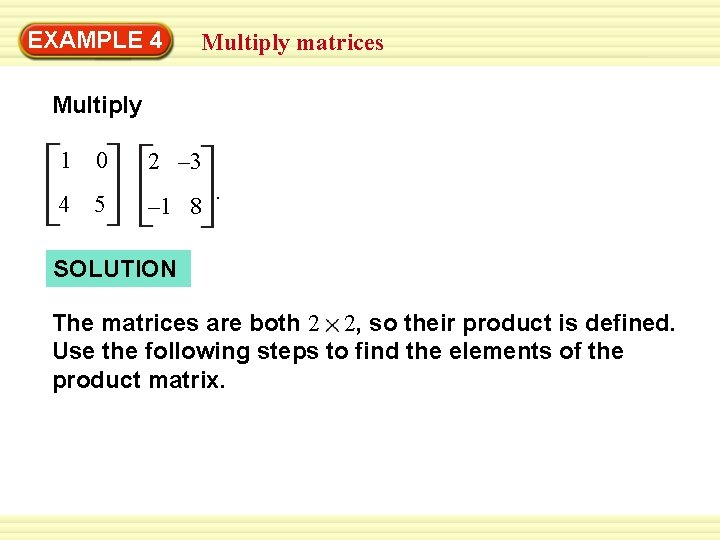EXAMPLE 4 Multiply matrices Multiply 1 4 0 5 2 – 3 – 1 8 . SOLUTION The matrices are both 2 2, so their product is defined. Use the following steps to find the elements of the product matrix.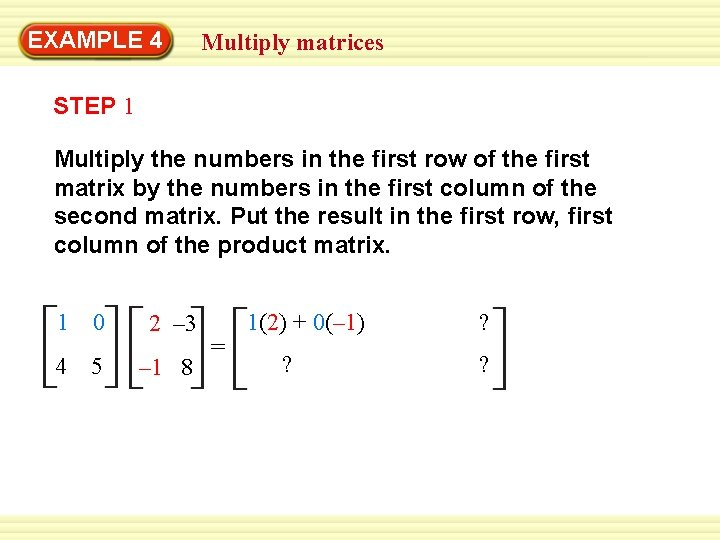EXAMPLE 4 Multiply matrices STEP 1 Multiply the numbers in the first row of the first matrix by the numbers in the first column of the second matrix. Put the result in the first row, first column of the product matrix. 1 0 2 – 3 4 5 – 1 8 = 1(2) + 0(– 1) ? ? ?EXAMPLE 4 Multiply matrices STEP 2 Multiply the numbers in the first row of the first matrix by the numbers in the second column of the second matrix. Put the result in the first row, second column of the product matrix. 1 0 2 – 3 4 5 – 1 8 = 1(2) + 0(– 1) 1(– 3) + 0(8) ? ?EXAMPLE 4 Multiply matrices STEP 3 Multiply the numbers in the second row of the first matrix by the numbers in the first column of the second matrix. Put the result in the second row, first column of the product matrix. 1 0 2 – 3 4 5 – 1 8 = 1(2) + 0(– 1) 1(– 3) + 0(8) 4(2) + 5(– 1) ?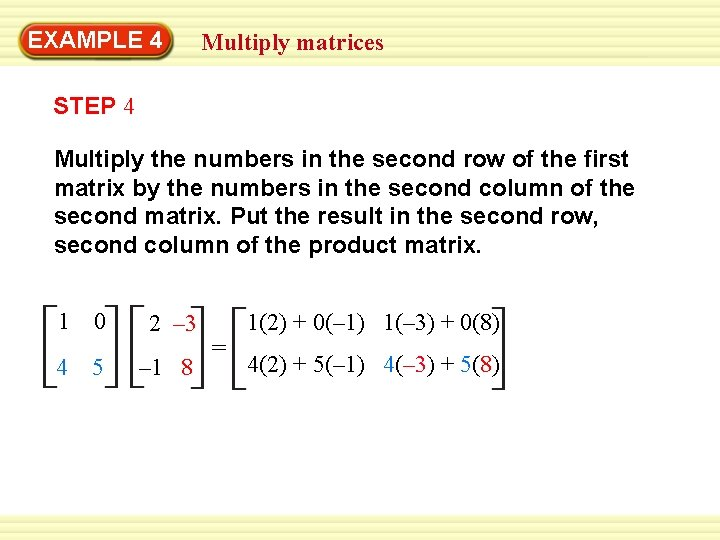EXAMPLE 4 Multiply matrices STEP 4 Multiply the numbers in the second row of the first matrix by the numbers in the second column of the second matrix. Put the result in the second row, second column of the product matrix. 1 0 2 – 3 4 5 – 1 8 = 1(2) + 0(– 1) 1(– 3) + 0(8) 4(2) + 5(– 1) 4(– 3) + 5(8)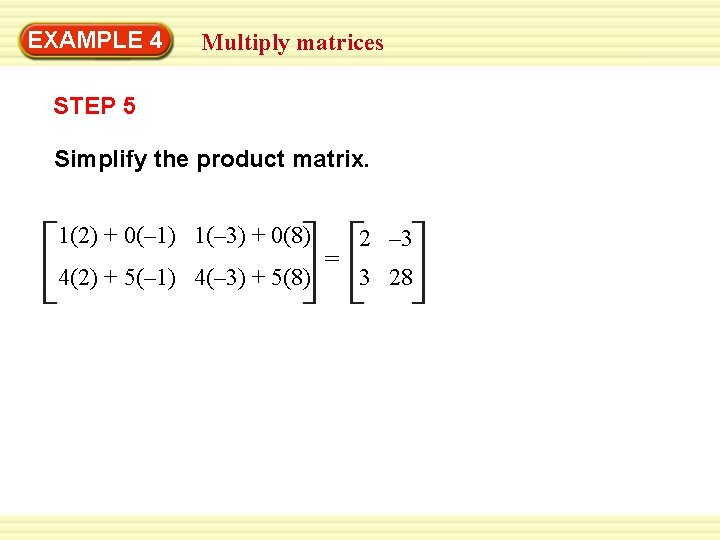EXAMPLE 4 Multiply matrices STEP 5 Simplify the product matrix. 1(2) + 0(– 1) 1(– 3) + 0(8) 4(2) + 5(– 1) 4(– 3) + 5(8) = 2 – 3 3 28EXAMPLE 5 Solve a real-world problem SOFTBALL Two softball teams submit equipment lists for the season. A bat costs \$20, a ball costs \$5, and a uniform costs \$40. Use matrix multiplication to find the total cost of equipment for each team.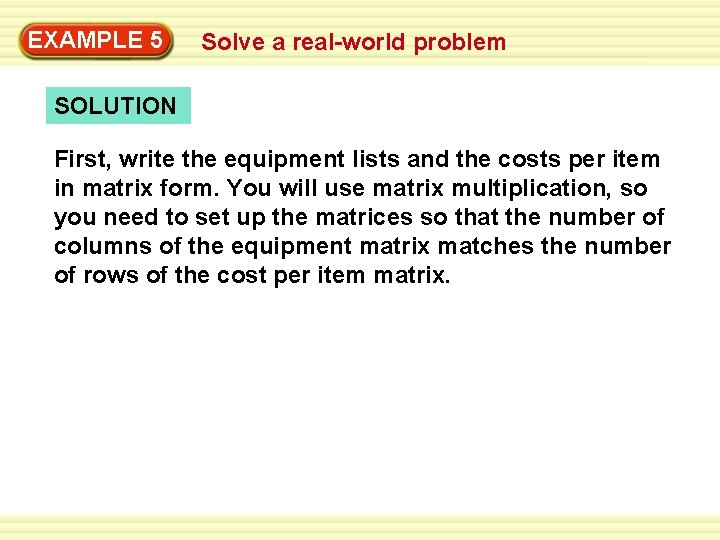EXAMPLE 5 Solve a real-world problem SOLUTION First, write the equipment lists and the costs per item in matrix form. You will use matrix multiplication, so you need to set up the matrices so that the number of columns of the equipment matrix matches the number of rows of the cost per item matrix.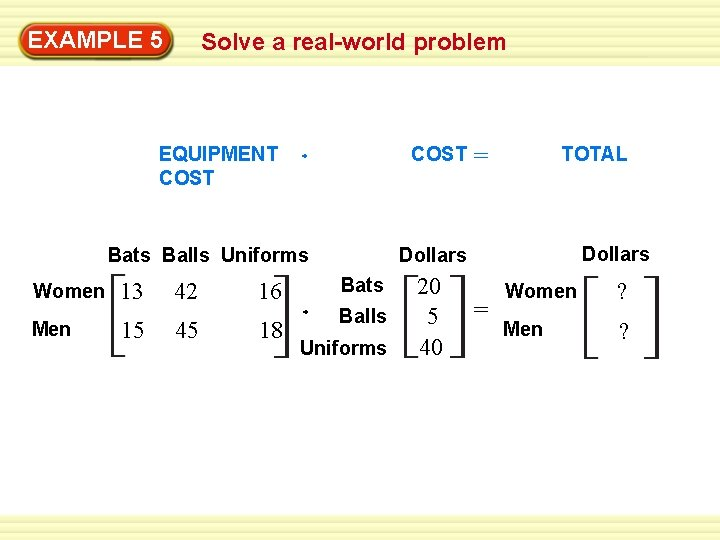EXAMPLE 5 Solve a real-world problem EQUIPMENT COST Bats Balls Uniforms Women Men 13 15 42 45 16 18 = TOTAL Dollars Bats Balls Uniforms 20 5 40 = Women ? Men ?EXAMPLE 5 Solve a real-world problem You can find the total cost of equipment for each team by multiplying the equipment matrix by the cost per item matrix. The equipment matrix is 2 3 and the cost per item matrix is 3 1, so their product is a 2 1 matrix. 13 42 16 15 45 18 20 5 40 = 13(20) + 42(5) + 16(40) 15(20) + 45(5) + 18(40) = 1110 1245 ANSWER The total cost of equipment for the women’s team is \$1110, and the total cost for the men’s team is \$1245.for Examples 4 and 5 GUIDED PRACTICE Use the matrices below. Is the product defined? Explain. A = – 3 4 B = [2 1] C = 6. 7 0 – 9. 3 5. 2 6. AB ANSWER Yes; the number of columns in A is equal to the number of rows in B.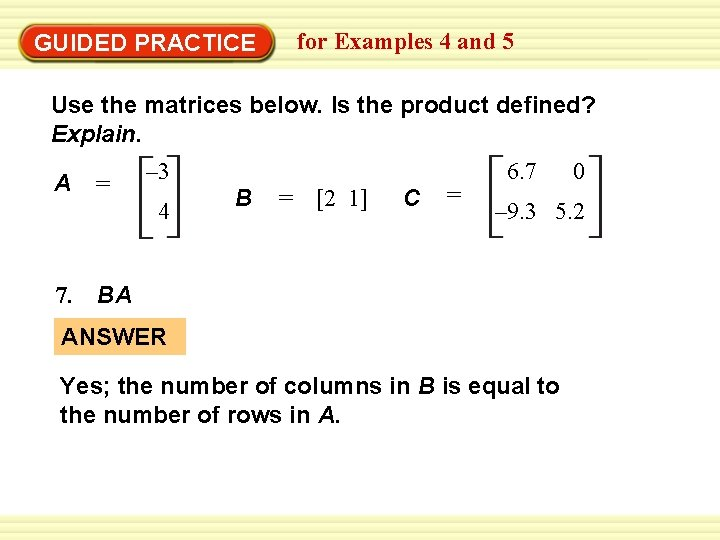for Examples 4 and 5 GUIDED PRACTICE Use the matrices below. Is the product defined? Explain. A = – 3 4 B = [2 1] C = 6. 7 0 – 9. 3 5. 2 7. BA ANSWER Yes; the number of columns in B is equal to the number of rows in A.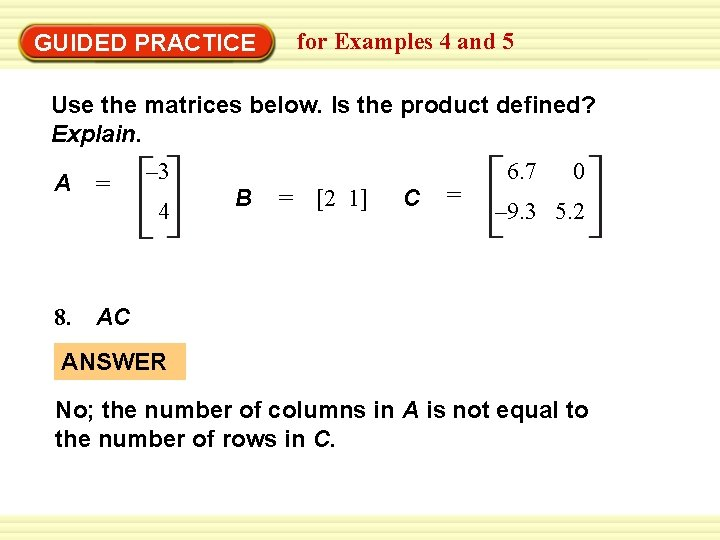for Examples 4 and 5 GUIDED PRACTICE Use the matrices below. Is the product defined? Explain. A = – 3 4 B = [2 1] C = 6. 7 0 – 9. 3 5. 2 8. AC ANSWER No; the number of columns in A is not equal to the number of rows in C.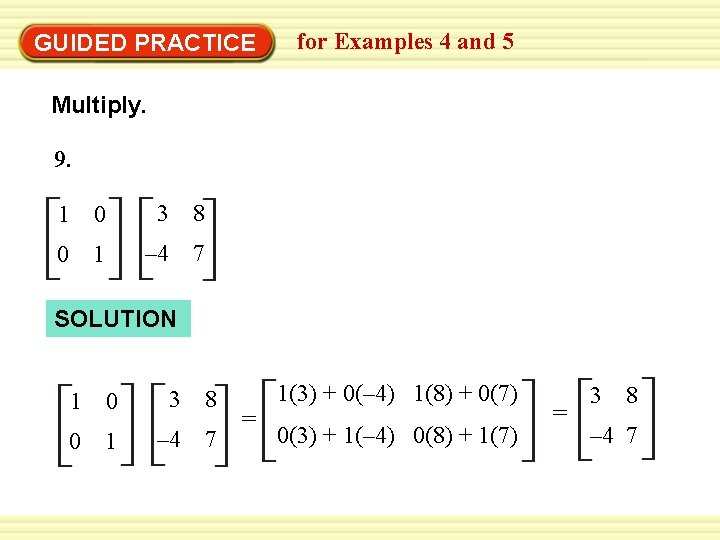GUIDED PRACTICE for Examples 4 and 5 Multiply. 9. 1 0 3 8 0 1 – 4 7 SOLUTION 1 0 3 8 0 1 – 4 7 = 1(3) + 0(– 4) 1(8) + 0(7) 0(3) + 1(– 4) 0(8) + 1(7) = 3 8 – 4 7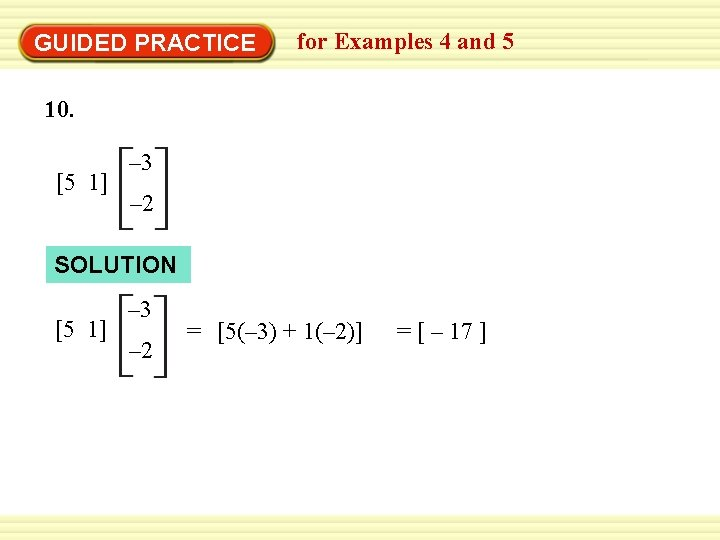GUIDED PRACTICE for Examples 4 and 5 10. [5 1] – 3 – 2 SOLUTION [5 1] – 3 – 2 = [5(– 3) + 1(– 2)] = [ – 17 ]GUIDED PRACTICE for Examples 4 and 5 11. 5 1 1 – 1 2 – 4 5 1 SOLUTION 5 1 1 – 1 2 – 4 5 1 = 5(2) + 1(5) 5(– 4) + 1(1) 1(2) – 1(5) 1(– 4) + – 1(1) = 15 – 19 – 3 – 5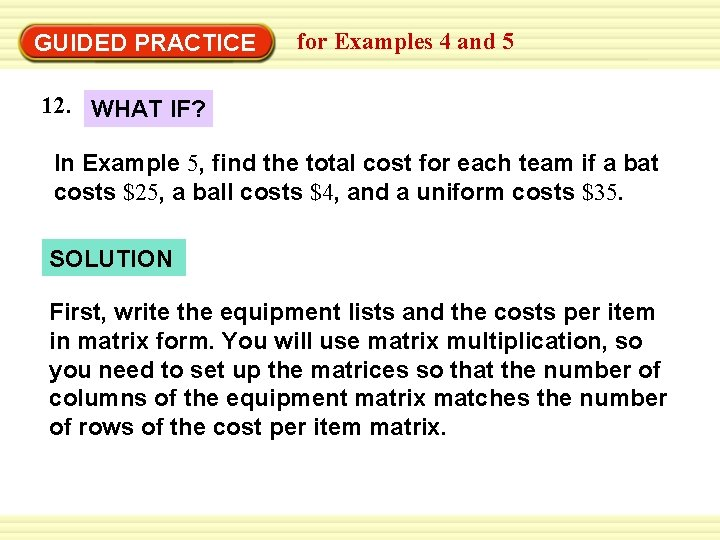GUIDED PRACTICE for Examples 4 and 5 12. WHAT IF? In Example 5, find the total cost for each team if a bat costs \$25, a ball costs \$4, and a uniform costs \$35. SOLUTION First, write the equipment lists and the costs per item in matrix form. You will use matrix multiplication, so you need to set up the matrices so that the number of columns of the equipment matrix matches the number of rows of the cost per item matrix.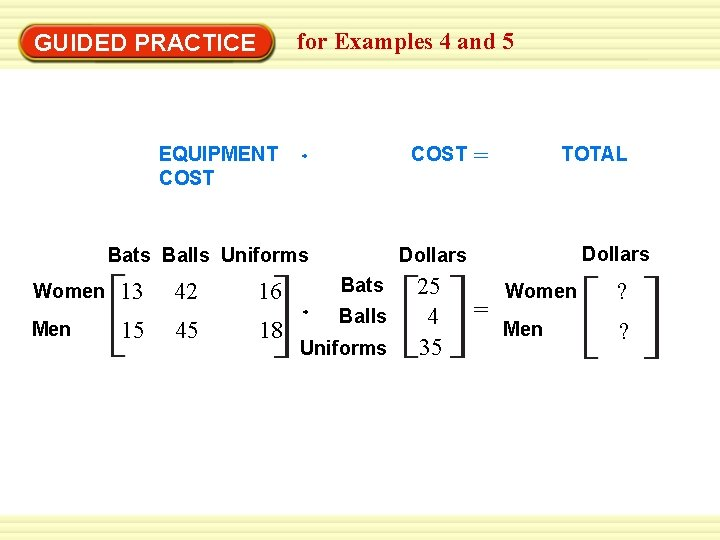for Examples 4 and 5 GUIDED PRACTICE EQUIPMENT COST Bats Balls Uniforms Women Men 13 15 42 45 16 18 = TOTAL Dollars Bats Balls Uniforms 25 4 35 = Women ? Men ?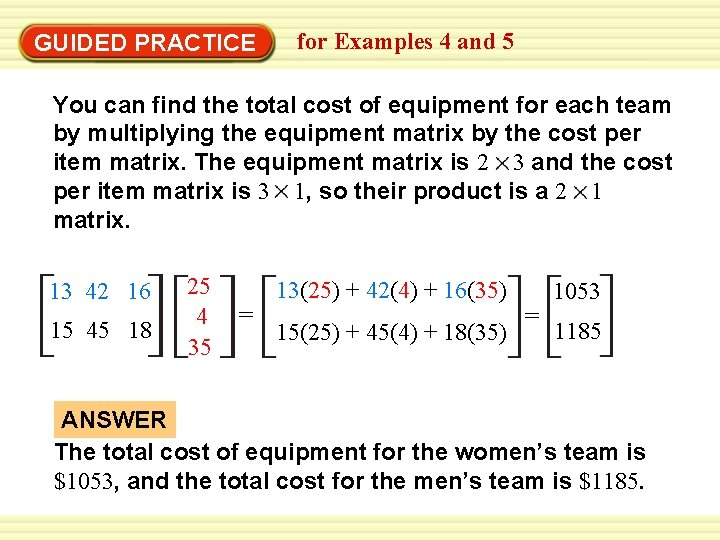GUIDED PRACTICE for Examples 4 and 5 You can find the total cost of equipment for each team by multiplying the equipment matrix by the cost per item matrix. The equipment matrix is 2 3 and the cost per item matrix is 3 1, so their product is a 2 1 matrix. 13 42 16 15 45 18 25 4 35 = 13(25) + 42(4) + 16(35) 15(25) + 45(4) + 18(35) = 1053 1185 ANSWER The total cost of equipment for the women’s team is \$1053, and the total cost for the men’s team is \$1185.